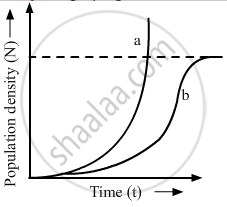# Study the Graph Given Below and Answer the Questions that Follow - Biology

Study the graph given below and answer the questions that follow :(i) Write the status of food and space in the curves (a) and (b).
(ii) In the absence of predators, which one of the two curves would appropriately depict the prey population?
(iii) Time has been shown on X-axis and there is a parallel dotted line above it. Give the significance of this dotted line

#### Solution

(i) There is ample food and space for the population depicted by curve (a). When the resources are unlimited, the curve is exponential. There are limiting food and space for the population depicted by curve (b). When the resources are limiting, the curve becomes sigmoid.

(ii) In the absence of predators, curve (a) would appropriately depict the prey population.

(iii) The dotted line represents the carrying capacity of the environment. The carrying capacity represents the size of population that the environment can hold by providing necessary resources. When a population reaches this line its population size is stabilised by various environmental factors.

Concept: Population - Population Attributes
Is there an error in this question or solution?

Share